# Test: Irrigation

## 25 Questions MCQ Test GATE Civil Engineering (CE) 2023 Mock Test Series | Test: Irrigation

Description
Attempt Test: Irrigation | 25 questions in 75 minutes | Mock test for GATE preparation | Free important questions MCQ to study GATE Civil Engineering (CE) 2023 Mock Test Series for GATE Exam | Download free PDF with solutions
*Answer can only contain numeric values
QUESTION: 1

### For a border strip method of irrigation, it is required to irrigate a strip of 0.04 Ha area from a tube well with a discharge of 0.02 cumec. The infilteration capacity of the soil may be taken as 5cm/hr. Calculate the maximum area that can be irrigated from this tubewell. (in Ha) (Important - Enter only the numerical value in the answer)

Solution: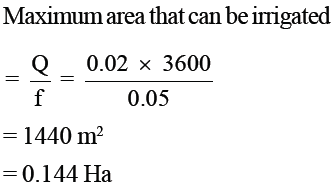QUESTION: 2

### During a particular stage of the growth of a crop, consumptive use of water is 28 mm/day. If the amount of water available in the soil is 25% of 80 mm depth of water, what is the frequency of irrigation?

Solution:

Water available to the soil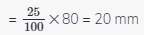= 80 – 20 = 60 mm

Frequency of irrigation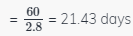Thus the crop should be irrigated after every 21 days.

QUESTION: 3

### In a canal irrigation project, 76% of the culturable command area (CCA) remained without water during kharif season and 58% of CCA remained without water during Rabi season in a particular year, Rest of the area got irrigated in each crop , respectively. What is the intensity of irrigation for the project in the year?

Solution:

Area irrigated under Rabi = 42%
Area irrigated under Kharif = 24%
Intensity of irrigation =66%

QUESTION: 4

The wave height , in meters , generated on the surface of a reservoir, having a fetch Length F=30km , due to wind blowing on the surface of the reservoir at a velocity of 30 km/hr is :

Solution: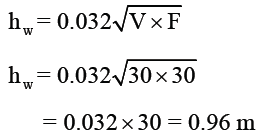QUESTION: 5

If the discharge required for different crops is 0.4 cumecs in the field and the capacity factor and time factors are 0.8 and 0.5 respectively. Then what is the design discharge of the distributary at its head?

Solution: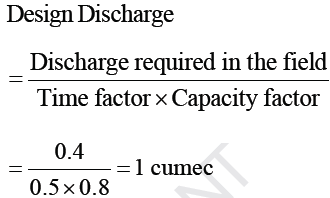QUESTION: 6

Determine the watering interval for field which can be irrigated using surface method of irrigation with a source of water having a discharge of 1000 liters / minutes. The available moisture holding capacity of the soil is 180mm per meter and depth of root zone is 1.1m. Irrigation is done when 40% of the available moisture is used. The total losses during irrigation are  35% and peak daily moisture use is 4.5mm _______

Solution: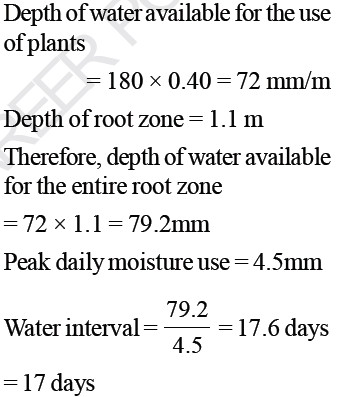*Answer can only contain numeric values
QUESTION: 7

Determine the net depth of irrigation required to irrigate the field 1000 m long and 10 m wide from a source supplying water at the rate of 30000 liters / hr in clay loam soil in moderate climate. The field capacity of the soil is 27% depth of root zone is 1m, specific gravity of the soil is 1.35 and irrigation is started when 50% of
the available moisture has been used _______ mm

(Important - Enter only the numerical value in the answer)

Solution: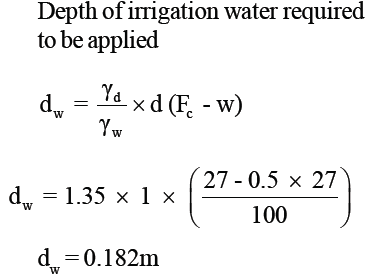QUESTION: 8

With the increase in quantity of water supplied the yield of most crops :

Solution:
QUESTION: 9

According to Lacey, depth of scour in a river depends upon the straightness of the reach. If D is the depth of scour in regime flow in a right angled bend, it is

Solution:
QUESTION: 10

For a 1m depth of flow in a canal with CVR equal to 0.9, according to Kennedy's theory the velocity of flow will be :

Solution: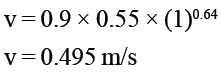*Answer can only contain numeric values
QUESTION: 11

A sandy loam soil holds water at 180 mm/m depth between field capacity and Permanent Witting point. The root zone depth of the crop is 80cm and allowable depletion of water is 34%. The area to be irrigated is 60Ha and water can be delivered at 28Lps. The surface irrgation application efficiency is 40%. Calculate the
time required (in Hrs) to irrigate 4Ha plot?

(Important - Enter only the numerical value in the answer)

Solution: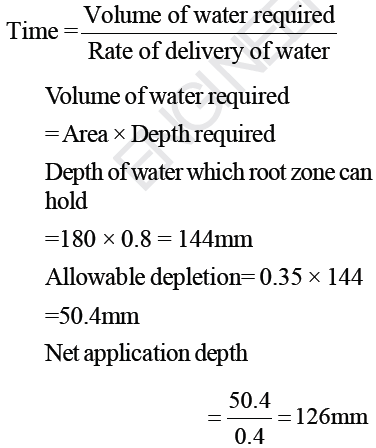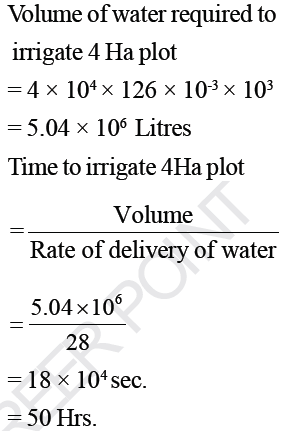QUESTION: 12

For border strip method of irrigation determine the time required to irrigate a strip of land of 0.08 Ha in area from a tube well  with a discharge of 0.02 cumec. The infilteration capacity of the soil may be taken as 6cm/hr and the average depth of flow on the field as 10cm.

Solution: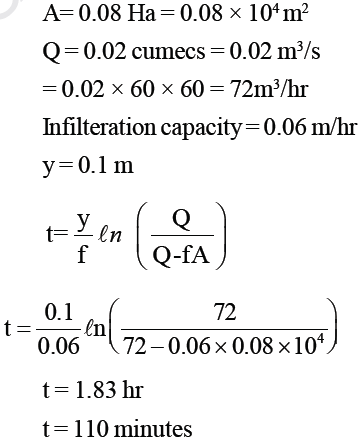QUESTION: 13

What is the regime scour depth for a channel in soil with silt factor of unity and carrying 8 m2/s of discharge intensity in accordance with Lacey’s regime theory?

Solution:

Regime scour depth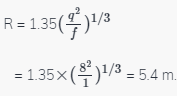QUESTION: 14

A soil sample has an exchangeable sodium percentage of 16% , its electrical conductivity is 3.2 millimhos/cm and pH of 9.5. How is the soil classified?

Solution:

In case of alkaline soil ESP > 15 and pH lies in range 8.5 - 10.0

QUESTION: 15

The total number of independent equations that form the Lacey regime theory is :

Solution:
QUESTION: 16

A canal was designed to supply the irrigation needs of 1200 hectares to land growing rice of 140 days base period having a Delta of 134cms. If this canal water is used to irrigate wheat of base period 120 days having a Delta of 52 cm, the area (in Hectares) that can be irrigated is

Solution: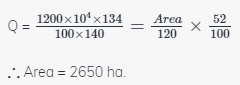QUESTION: 17

Garret’s diagram is used to :

Solution:
QUESTION: 18

Water flowing at a rate of 10 m3/s is diverted to a 32 Ha field for 4h. Soil probing often irrigation showed that  0.3m of water had been stored in the root zone. Water application efficiency in this case would be :

Solution: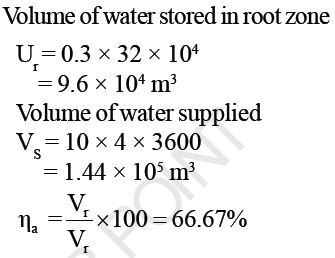QUESTION: 19

Sodium Adsorption Ratio is expressed as :

Solution:
QUESTION: 20

The width of a dowla is generally kept between 30 to 60 cm and its height above the road level should invariably be more than

Solution:
*Answer can only contain numeric values
QUESTION: 21

A water course commands an irrigated are 1000 hectares. The intensity of irrigation of rice in this area is 70%. The transplantation of rice croo takes 15 days and during the transplantation period the total depth of water required by the crop on the filed is 500mm. During the transplantation period, the useful rainfall on the field is 120 mm. Find the duty of irrigation water for the crop at the head of water course assuming losses of water to be 20% in the water course _____ hectares/ cumec

Solution:

Depth of water required on the field during transplantation = 500 mm

Useful rainfall during this period = 120 mm

Depth of water required to be supplied by the water Course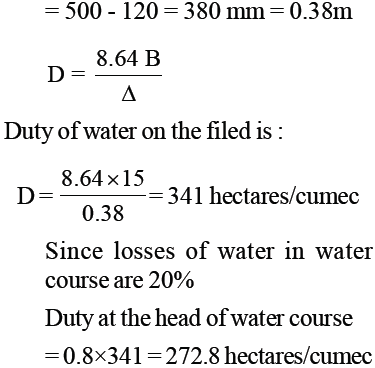*Answer can only contain numeric values
QUESTION: 22

The field capacity of a certain soil is 12.6% and specific gravity is 1.42. If the moisture content present in the soil before irrigation is 8.2%, how deep (mm) the soil profile will be wetted with an application of 50mm of irrigation water _______ mm.

(Important - Enter only the numerical value in the answer)

Solution: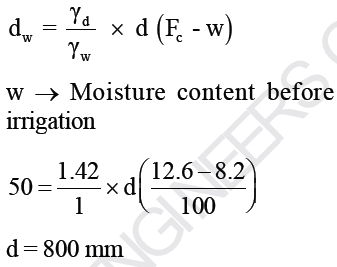QUESTION: 23

Borrow pits should preferably be located in

Solution:
QUESTION: 24

Lacey's regime scour depth is given by :

Where q is the discharge per unit width and f is the silt factor

Solution:
QUESTION: 25

Wetted perimeter of a regime channel for a discharge of 64 cumec as per Lacey's theory will be :

Solution: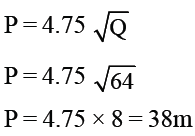Use Code STAYHOME200 and get INR 200 additional OFF Use Coupon Code